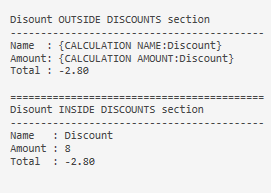# Weird Discount behaviour outside of [DISCOUNTS] section

Hi,

Apparently it is not possible to use
`CALCULATION NAME:Discount` or CALCULATION AMOUNT:Discount
outside the DISCOUNT section, no values returned.
However
`CALCULATION TOTAL:Discount` returns a value.

Is it a bug or is there a valid reason to it?

Printer template:

``````[LAYOUT]

<L00>Disount OUTSIDE DISCOUNTS section
<F>-
<L00>Name  : {CALCULATION NAME:Discount}
<L00>Amount: {CALCULATION AMOUNT:Discount}
<L00>Total : {CALCULATION TOTAL:Discount}
<L00>
<F>=
<L00>Disount INSIDE DISCOUNTS section
<F>-
{DISCOUNTS}

[DISCOUNTS]
<L00>Name   : {CALCULATION NAME}
<L00>Amount : {CALCULATION AMOUNT}
<L00>Total  : {CALCULATION TOTAL}
``````

Result:Using
SambaPOS App 5.2.7 2018-07-30 11:12 DB:143-143 SQ
Not touched any Discount or calculation default setting, nor with action, nor with script.
Making the discount in settle screen, clicking Discount %

Are they meant to be available outside section? Name would seem pretty pointless outside section as it would need the name specified in the expression if it were anyway…

1 LikeYes name would be pointless !

But not amount …

I think I already explain to you what I am trying to acheive: writing ONE line to a file.
That line contains 32 fields, some of them about discount information.

It works if i use something like that:

``````<L00>field 1|field 2|.......|{DISCOUNTS}| ....|field 32

[DISCOUNTS]
the correct format and calculation
``````

Unfortunatelly, the line format is more like that:

`<L00>field 1|field 2|.......|discount %|field 6|field 7|{ENTITIES}|Discount in money| ..|Total - Discount - VAT|...|field 32`

(no, I cannot modify the file structure)

What’s the difference between amount and total if your specifying the calculation name?

For discount,

• amount is the % (in %) of the discount, here: 8 (%)
• total is the discount on that bill in money, here : \$-2.80 (also I need it positive for the file)

Only `{CALCULATION TOTAL:X}` is available at ticket level, the rest can only be used under calculation section (`[DISCOUNTS]` or `[SERVICES]`).

You can check the template help to the right of the printer template, it clearly shows this.

3 Likes

`CALCULATION TOTAL:X Calculation Total`

At least that clear … no need to waste more time trying to make it work1 Like